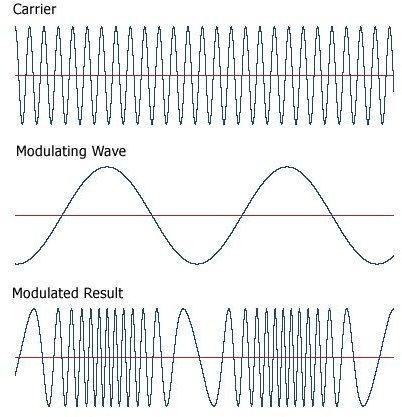# FM concepts explained – What is frequency modulation?

## FM Concepts

Before we start discussing FM modulation we take a look at some important terms.

Modulation: The process by virtue of which some characteristic, normally amplitutude, frequency, phase of a voltage signal is varied in accordance to the instantaneous value of some other voltage signal called “Carrier”.

Need of modulation: For successful transmission and reception of information (Audio signal, video signal, code) this process is essential. Audio and video signal cannot be sent directly from broadcast station for thousand of miles, as these signals have low frequency and low power. The signal strength degrades as it propagates due to various atmosphere effects. Some of other main reasons of modulation are:

1. If every body start sending low frequency signal their mutual interference can take place.
1. For a proper reception of signal the size of antenna should be of the order of the wave length (λ) of the signal to be received. So lower the frequency of signal greater will be its wave length (as λ ∞ 1/ f) and much larger antenna will be required and vice versa.

Frequency Modulation (FM)

It is a process in which the frequency of the carrier is varied in accordance with the instantaneous value of modulating voltage. The amount of change in frequency is determined by the amplitude of the modulating signal. As seen in fig, frequency of the carrier signal increase as the amplitude of the modulating signal rises while decreases as the amplitude of the modulating voltage drops down. When the amplitude of the modulating signal is zero the carrier signal has frequency at its normal value “fo” called resting or center frequency.

• The amounts of frequency variation (frequency deviation or shift) depend on the amplitude of the audio signal. Greater the audio signal, higher will be the frequency shift and vice versa. According to the international law regarding frequency spectrum the maximum frequency deviation should be 75 KHz.

The rate of frequency deviation depends on signal frequency.The total variation in frequency from lowest to highest called Carrier swing (CS), obviously the carrier swing =2 * frequency deviation. We know that max deviation of 75Hz is allowed for commercial FM broadcast stations in range 88-168MHz within VHF band, hence FM channel width =2 * 75 =150Hz,with a guard band of 25KHz on either side. The channel width become 2(75 +25) =200 KHz. The purpose of guard band is to prevent a signal from its adjacent channel interference.

Modulation index: It is given by the expression: Frequency deviation/Modulating frequency =∆f/fm.

Deviation Ratio: In the modulation index equation if we allow max frequency deviation and max modulating frequency then it become “Deviation

Ratio” Deviation ratio = (∆f) max /fm (max).

Advantages of FM modulation

Both FM and AM are practical system but differ in performance and characteristics:

1. FM system is more immune to noise so higher signal to noise ration (S/N)
2. Due to guard band interference from adjacent channel does not take place.
3. All the transmitted power in FM is useful, while in AM most of the power in carrier and useless.

Drawbacks of FM modulation

1) Required much wider band almost 7-15 times the AM.

2) Sophisticated and expensive equipment are required.

3 )Coverage area for the FM is reserved, while the only hindrance to AM is line of sight (LOS) problem

## Images